$$\require{cancel}$$

# 5.2: Rotational Inertia

## Rotational Kinetic Energy and Rotational Inertia

While our first approach to studying dynamics for linear motion was Newton's laws (forces cause accelerations), we will find it easier to examine rotational dynamics from a standpoint of energy first. Consider an object that is rotating around a stationary center of mass. Does such an object possess kinetic energy? We might be inclined to say that it does, but with the center of mass not moving, its momentum is zero, which would make the quantity $$\frac{p^2}{2m}$$ also equal to zero.

We must not be such slaves to memorized equations! This equation (by itself) never applied to a system of multiple particles, which can easily have a zero total momentum and yet still have a nonzero kinetic energy. Well, rigid objects are systems of multiple particles, and when they are rotating, all those particles (except those right at the pivot point) are moving, which means they all have kinetic energy. At any given moment, there are particles moving in opposite directions, and if the center of mass of the object is stationary, these opposite momenta (which are vectors) cancel, Their kinetic energies, on the other hand, are not vectors, and are all positive numbers, so they can never cancel out.

In some sense, the particles comprising a rotating object can be thought of as contributing to the "internal" energy of the object as we discussed back in Section 4.4. But doing this runs contrary to the main reason for the introduction of the mechanical/internal energy idea, which was to separate the kinetic energy of the system that we can clearly see from the kinetic energy that is concealed from us inside the confines of the system. We can clearly see rotational motion of an object, so we choose to include rotational kinetic energy in the category of "mechanical energy."

Okay, so a rotating object does possess kinetic energy. Our task now is to express that kinetic energy in terms of the rotation variables we have already defined, but all we know about kinetic energy is the linear version. In Figure 5.2.1 we consider the motion of a single particle within a rigid rotating object.

Figure 5.2.1 – Motion of a Single Particle in a Rotating Rigid Body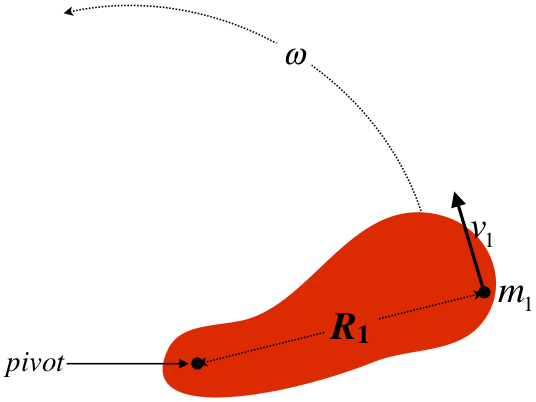This is particle #1 – one of many within the rigid object. We can write down its kinetic energy, and in fact we can express it in terms of a rotational variable and the particle's distance from the pivot:

$KE_1 = \frac{1}{2}m_1v_1^2 = \frac{1}{2}m_1\left(R_1\omega\right)^2 = \frac{1}{2}m_1R_1^2\omega^2$

If we want the total kinetic energy of the object, we need to add up the kinetic energy of all the particles. Thanks to our definition of angular velocity, we can factor that part out of all the terms:

$KE_{whole\;object} = \frac{1}{2}m_1v_1^2 + \frac{1}{2}m_2v_2^2 + \dots = \frac{1}{2}m_1R_1^2\omega^2 + \frac{1}{2}m_2R_2^2\omega^2 + \dots = \frac{1}{2} \left[ m_1R_1^2 + m_2R_2^2 + \dots \right] \omega^2$

Notice that the quantity in brackets in the final equality is determined by the distribution of mass throughout the object. That is, it is an intrinsic property of the object, not dependent upon how it is moving. We generally abbreviate this quantity with an $$I$$, which gives us a familiar form for the kinetic energy formula:

$KE_{rotating\;rigid\;body} = \frac{1}{2}I\omega^2, \;\;\;\;\;\;\;\;\; where:\;\; I \equiv m_1R_1^2 + m_2R_2^2 + \dots$

This looks just like the linear kinetic energy formula, with the angular speed replacing the linear speed, and $$I$$ replacing the mass. This quantity certainly contains some information about the mass of the object, but it is more complicated than just the mass, and is called the rotational inertia, or more commonly (and less descriptively), the moment of inertia. Notice that this “inertia” depends not only upon the amount of stuff (mass), but also where that mass is. This means that two different objects can actually weigh exactly the same amount, but when they are rotated at equal speeds, one of them has more KE than the other. As you might guess, this occurs when more of the mass is concentrated farther from the pivot for the former object than the latter.

It is important to note that we will only be considering rotations around axes, not points. In our two-dimensional figures, an axis that is perpendicular to the plane of the figure is indistinguishable from a single point, but we will not discuss motion that involves an object's motion changing its plane of rotation. So rotational inertia for three-dimensional objects involves the distances of the tiny masses from a common axis, not a common point.

## Calculating Rotational Inertia for Continuous Objects

Our task is to compute the rotational inertia, for which the formula in terms of masses and their positions is different from the one for center of mass (see Section 4.2), but the procedure is exactly the same. We start with the same picture (Figure 4.2.3, which is reproduced below), and convert the sums into integrals, as before.

Figure 5.2.2 – Setup Diagram for Computations Involving Mass Density of a Thin Rod$I = dm_1x_1^2 + dm_2x_2^2 + \dots = \int\limits_{x=0}^{x=L} dm\;x^2$

Note that the rotational inertia is calculated around a specific pivot point, which we have chosen to be our origin for the calculation.

As before, we replace the $$dm$$ with $$\lambda \left(x\right) dx$$, and we have our formula for the rotational inertia along the $$x$$-axis around the pivot point at the origin:

$I = \int\limits_{x=0}^{x=L} \lambda\left(x\right)x^2dx$

Let's return to the cases for which we computed the centers of mass in Section 4.2 – the uniform and non-uniform rod. Unlike the case of center of mass, where the answer is a location on the rod, the final answer for the rotational inertia will have units of $$kg \cdot m^2$$, and the formula for it will involve the total mass of the rod and its length. Also it is important to remember that while the center of mass is a location that doesn't depend upon where we put our coordinate system to calculate it, the rotational inertia is only defined relative to a specific pivot point.

### A Uniform Rod of Mass M and Length L, Pivoted About an End

Plugging the constant $$\lambda$$ into Equation 5.2.5 and performing the integral gives:

$I = \int\limits_{x=0}^{x=L} \lambda x^2dx = \lambda \left[\frac{1}{3}x^3\right]_0^L =\frac{1}{3} \lambda L^3$

We are not finished yet, because this answer is not in terms of the rod's mass. Since this rod is uniform, the mass is simply the (constant) density multiplied by its length, which gives:

$I =\frac{1}{3} \left(\dfrac{M}{L}\right) L^3 = \frac{1}{3} M L^2$

We will find that every rotational inertia we encounter has this basic form: A constant (usually written as a fraction) multiplied by the mass of the object and the square of some natural length dimension of the object. In this case it is the length of the rod, but it may also be something like the radius of a disk or sphere.

### A Non-Uniform Rod of Mass M and Length L, Pivoted About Its Lighter End

Now we repeat the process for the non-uniform density function for which we computed the center of mass in Section 4.2:

$\lambda\left(x\right) = \lambda_o\left(\dfrac{x}{L}+1\right)$

Note that unlike the uniform case, the results should not come out the same for both ends of the rod, since more of the mass is concentrated near the end at $$x=L$$. We are calculating this rotational inertia about the lighter end, since all of the $$x$$ values in the integral are measured from that end.

$I = \int\limits_{x=0}^{x=L} \lambda_o\left(\dfrac{x}{L}+1\right)x^2dx = \lambda_o \left[\dfrac{1}{4L}x^4 +\frac{1}{3} x^3\right]_0^L = \frac{7}{12} \lambda_o L^3$

We are not done yet, because we are given the mass of the rod, not the constant $$\lambda_o$$. We therefore need to compute the total mass in terms of this constant. We do this by integrating density function over the length of the rod:

$M = \int\limits_{x=0}^{x=L} \lambda_o\left(\dfrac{x}{L}+1\right)dx = \lambda_o \left[\dfrac{1}{2L}x^2 + x\right]_0^L = \frac{3}{2} \lambda_o L \;\;\; \Rightarrow \;\;\; \lambda_o = \frac{2}{3} \dfrac{M}{L}$

Plugging this back in above gives our answer:

$I = \frac{7}{12} \left(\frac{2}{3} \dfrac{M}{L}\right) L^3 = \frac{7}{18}ML^2$

Example $$\PageIndex{1}$$

Find the rotational inertia of the non-uniform rod of mass $$M$$ and length $$L$$ whose mass density function is given by Equation 5.2.8, when rotated about its heavier end ($$x=L$$).

Solution

The difference between this calculation and the one above is that the variable $$x$$ that appears in Equation 5.3.5 doesn't match the $$x$$ that appears in the density formula. The density formula is referenced to our coordinate system, but the $$x$$ in the rotational inertia integral represents the distance of each tiny piece of mass $$dm$$ from the pivot point at $$x=L$$. So we need to make a change in the integral so that the $$x$$ variable that appears in it matches the $$x$$ in the density function. Making the substitution $$x \rightarrow L-x$$ (so $$dx \rightarrow -dx$$), into the integral does the trick, because then the integrand is zero at the pivot point ($$x=L$$) as it should be:

$\begin{array}{l} I_{heavy\;end} & = \int\limits_{x=L}^{x=0} dm\;\left(x-L\right)^2 = \int\limits_{x=L}^{x=0} \lambda\left(x\right)\left(x-L\right)^2\left(-dx\right)\ = \int\limits_{x=0}^{x=L} \lambda_o\left(\dfrac{x}{L}+1\right)\left(x-L\right)^2dx \\ & = \lambda_o \int\limits_{x=0}^{x=L} \left(\dfrac{x^3}{L}-x^2-xL+L^2\right)dx = \lambda_o\left[\dfrac{x^4}{4L}-\dfrac{x^3}{3}-\dfrac{x^2L}{2}+xL^2\right]_0^L \\ & = \frac{5}{12}\lambda_o L^3 \end{array}\nonumber$

We need to plug in for $$\lambda_o$$ (which was computed above) to get our final answer:

$I_{heavy\;end} = \frac{5}{12}\left(\frac{2}{3} \dfrac{M}{L}\right) L^3 = \boxed{\frac{5}{18}ML^2} \nonumber$

## Principal Axes

It’s clear that the choice of the pivot is important to the calculation of the rotational inertia, but so is the axis. Real objects are 3-dimensional, so they actually have 3 independent rotation axes, each of which has its own rotational inertia around it. These axes are called the principal axes. The origin of these axes is located at – what else? – the center of mass of the object. The principal axes are only easy to identify for objects with some degree of symmetry. Some objects are so symmetric that more than one set of axes will work. For example, a uniform sphere has so much symmetry that any set of three mutually perpendicular axes whose origin coincides with the center of the sphere will work, and of course the rotational inertias around all these axes are the same.

The reason it is natural to define the origin of the principal axes to be at the center of mass is that if an object is rotating freely in space with no forces on it, its axis of rotation must pass through its center of mass (though it doesn't need to be around one of the principal axes). This is actually surprisingly easy to prove. Suppose an object was rotating around an axis that does not pass through the center of mass. This would mean that the center of mass is moving in a circle around the axis of rotation. But circular motion is accelerated motion. According to Newton's second law, the center of mass cannot be accelerating if there are no forces on the object, which contradicts our assumption.

## Computing Rotational Inertia Without Integration

Throughout our study of mechanics, our goal has been to develop shortcut tools to help us deal with physical systems in simpler ways. We developed work-energy so that we could solve problems that pay no attention to direction or time without slogging through Newton's laws (such as speed at a given height on a loop-de-loop). We developed impulse-momentum so that we could more easily solve problems involving systems in which the internal forces are complicated (such as collisions). Now we are developing a tools related to rigid body rotations so that we don't have to track the linear motions of all the particles in the system. With this very practical mindset, it is not surprising that physicists have developed tools for computing rotational inertia that avoid the ugliness of always having to perform integrals. The first such shortcut is simply a collection of rotational inertias that are associated with common symmetric geometries, such as rods, disks, and spheres. Our collection is given at the end of the section. There are two tools that we can combine with our collection of rotational inertias that will allow us to "bootstrap" our way to determining many more.

### Additivity Around a Common Axis

Suppose we know the rotational inertias of two separate objects around a common axis. If these two objects are attached so that they rotate together rigidly around that common axis, then the rotational inertia of the combined object is simply the sum of their rotational inertias. This is evident from the formula for rotational inertia: Each object has its own sum of $$mx^2$$ terms, and when the objects are combined such that their $$x$$ axes are common, then the new sum of $$mx^2$$ terms is simply the combination of the two individual sums. To summarize:

$I = I_1 +I_2$

Example $$\PageIndex{2}$$

Use the additive property of rotational inertia and the result given by Equation 5.2.7 to find the rotational inertia of a uniform thin rod of mass $$M$$ and length $$L$$ about its center of mass.

Solution

We can treat a rod rotated around an axis through its center as if it is two separate half-rods of half the mass and half the length, attached at their ends. The axis that passes though the center of the rod passes through the ends of these two half-rods, and we know the rotational inertia of each half-rod. The additivity property than gives us the rotational inertia of the whole rod about its center:

$I_{uniform\;thin\;rod\;about\;its\;center} = 2I_{half-rod\;about\;end} = 2 \left[ \frac{1}{3} \left( \dfrac{M}{2} \right) \left( \dfrac{L}{2} \right)^2 \right] = \boxed{ \frac{1}{12}ML^2} \nonumber$

### Parallel Axis Theorem

As we have seen multiple times already, just changing the axis around which an object is rotated will result in a different rotational inertia. Suppose we calculate the rotational inertia of an object about an axis, then slide that axis in a parallel fashion on the object, and calculate the new rotational inertia, then do it over and over, recording the new values each time. One might ask, "Where is the axis (parallel to the original one) for which the rotational inertia is the smallest?" Is there any way to guess where this might be, and is it unique, or might there be multiple places where the rotational inertia hits a minimum?

To answer this question, let's look at a one-dimensional object that lies along the $$x$$-axis, and consider its rotational inertia around the $$y$$-axis. Writing it as a sum rather than an integral, it is:

$I = m_1x_1^2 + m_2x_2^2 + \dots$

Now let's suppose we decide to change where we place the origin, moving it a distance $$+x$$ along the $$x$$-axis. When we do this, the distance from the axis to mass $$m_1$$ changes from $$x_1$$ to $$x_1-x$$. Also, since the original axis went through the origin, this new axis is no longer the $$y$$-axis – now it intersects the $$x$$-axis at $$x$$. The new rotational inertia is, therefore:

$I = m_1\left(x_1-x\right)^2 + m_2\left(x_2-x\right)^2 + \dots$

We can consider this to be a function of $$x$$. That is, this formula provides the rotational inertia of the object about the axis located at $$x$$. We can now answer our question about where the rotational inertia is a minimum by using calculus. The value of $$x$$ for which the function $$I\left(x\right)$$ is a minimum satisfies:

$0 = \dfrac{dI}{dx} = -2m_1\left(x_1-x\right) - 2m_2\left(x_2-x\right) + \dots$

Solving for $$x$$ here provides a familiar result:

$x = \dfrac{m_1x_1 + m_2x_2 + \dots}{m_1 + m_2 + \dots}$

The rotational inertia of an object for all axes parallel to each other is a minimum for the axis that passes through the center of mass! Actually, this should not be too surprising. The rotational inertia of an object will be minimized around an axis that is as close as possible to as much of the object's mass as possible, and the center of mass is the "average location of mass," so it makes sense that this would be "as close to as much of the object's mass as possible."

Given this information, we can write the rotational inertia of an object around an axis parallel to an axis passing through the center of mass a positive-valued "adjustment" to the rotational inertia around the center of mass. It turns out (we will not prove it here) that this adjustment is quite simple – it is just the mass of the object multiplied by the square of the offset distance between the new axis and the axis through the center of mass. This is called the parallel axis theorem:

$I_{new} = I_{cm} + Md^2,$

where $$d$$ is the distance separating the new axis and the center of mass.

Example $$\PageIndex{3}$$

Use the parallel axis theorem and the result given by Equation 5.2.7 to find the rotational inertia of a uniform thin rod of mass $$M$$ and length $$L$$ about its center of mass.

Solution

The distance that the end of the rod is separated from the rod's center of mass is $$d=L/2$$. Plugging this into the parallel axis theorem gives our answer, which agrees with what we got in Example 5.2.2:

$I_{new} = I_{cm} + Md^2 \;\;\; \Rightarrow \;\;\; I_{cm} = I_{new} - Md^2 = \frac{1}{3}ML^2 - M\left(\dfrac{L}{2}\right)^2 = \left(\frac{1}{3}-\frac{1}{4}\right)ML^2 = \boxed{\frac{1}{12}ML^2} \nonumber$

## Rotational Inertias of Some Common Geometries

In all of the cases indicated below, the mass of the object is $$M$$, and the material making up the object has uniform density. The reader is encouraged (as an exercise) to navigate their way between various relations using the additivity and parallel axes theorem tools. [Note: When it comes to rotating two-dimensional objects such as rings and disks, we will confine our studies to axes perpendicular to the two-dimensional planes in which these objects lie. For rotations around axes parallel to this plane, one would need yet another useful tool, known as the perpendicular axes theorem.]

### Thin Rods

Figure 5.2.3 – Thin Straight Rod Rotated About One end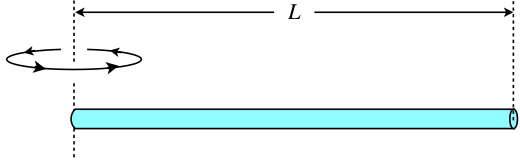$I = \frac{1}{3}ML^2$

Figure 5.2.4 – Thin Straight Rod Rotated About Center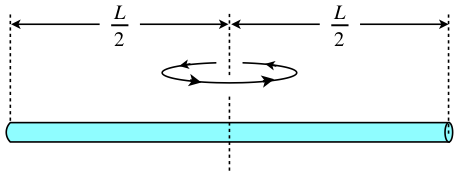$I = \frac{1}{12}ML^2$

Figure 5.2.5 – Thin Circular Ring (or Thin Cylindrical Shell) Rotated About Center$I = MR^2$

Figure 5.2.6 – Thin Circular Ring (or Thin Cylindrical Shell) Rotated About Edge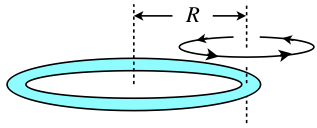$I = 2MR^2$

### Disks (or Cylinders)

Figure 5.2.7 – Solid Disk (or Cylinder) Rotated About Center$I = \frac{1}{2}MR^2$

Figure 5.2.8 – Solid Disk (or Cylinder) Rotated About Edge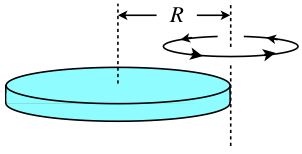$I = \frac{3}{2}MR^2$

Figure 5.2.9 – Hollow Disk (or Cylinder) Rotated About Center$I = \frac{1}{2}M\left(R_1^2+R_2^2\right)$

### Spheres

Figure 5.2.10 – Solid Sphere Rotated About Center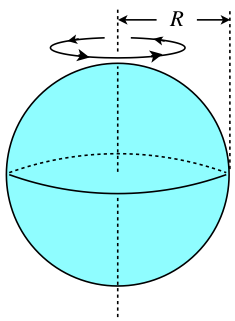$I = \frac{2}{5}MR^2$

Figure 5.2.11 – Solid Sphere Rotated About Edge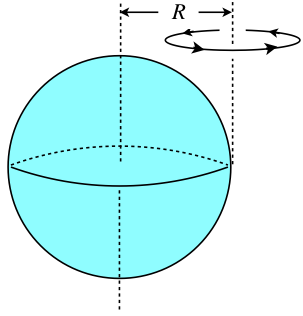$I = \frac{7}{5}MR^2$

Figure 5.2.12 – Thin Spherical Shell Rotated About Center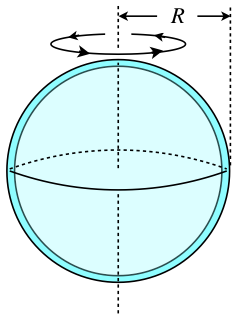$I = \frac{2}{3}MR^2$

Example $$\PageIndex{4}$$

The frame of a badminton racquet is constructed from two identical thin aluminum rods of uniform density, mass M, and length L. One of the rods is bent into a circle and is welded to the end of the other rod. Find the rotational inertia of this racquet around the axis perpendicular to the plane of the circle that passes through the point where the loop connects to the rod.

SolutionThe points labeled $$cm_1$$ and $$cm_2$$ are the centers of mass of the two rods, respectively. We have the rotational inertias of the two rods about their respective centers of mass, and using the fact that the length of the bent rod is its circumference gives:

$\begin{array}{l} I_{cm_1} = \dfrac{1}{12} ML^2 \\ I_{cm_2} = MR^2 = M\left(\dfrac{L}{2\pi}\right)^2 = \dfrac{1}{4\pi^2}ML^2 \end{array} \nonumber$

Now we need to use our two tools. First, we need to change the axes for each of these rotational inertias from $$cm_1$$ and $$cm_2$$ to the pivot. We do this using the parallel axis theorem. The distance that the new axis is from $$cm_1$$ is \frac{L}{2}, and the distance between $$cm_2$$ and the pivot is $$R=\frac{L}{2\pi}$$, so:

$\begin{array}{l} I_1 = I_{cm_1} + Md_1^2 = \dfrac{1}{12} ML^2 + M\left(\dfrac{L}{2}\right)^2 = \dfrac{1}{3} ML^2 \\ I_2 = I_{cm_2} + Md_2^2 = \dfrac{1}{4\pi^2}ML^2 + M\left(\dfrac{L}{2\pi}\right)^2 = \dfrac{1}{2\pi^2} ML^2 \end{array} \nonumber$

And now that we have the two rotational inertias about the same axis, we can use the additive property to get the total rotational inertia:

$I = I_1 + I_2 = \boxed{\left(\dfrac{1}{3} + \dfrac{1}{2\pi^2}\right)ML^2} \nonumber$